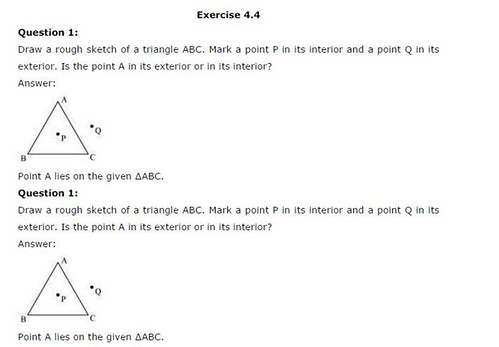# NCERT Solutions For Class 6 Maths Basic Geometrical Ideas Exercise 4.4

NCERT Solutions For Class 6 Maths Basic Geometrical Ideas Exercise 4.4

### NCERT Solutions For Class 6 Maths Basic Geometrical Ideas Exercise 4.4

NCERT Solutions for Class 6 Maths Chapter 4 Basic Geometrical Ideas Ex 4.4

Exercise 4.4

Ex 4.4 Class 6 Maths Question 1.
Draw a rough sketch of a triangle ABC. Mark a point P in its interior and a point Q in its exterior. Is the point A in its exterior or in its interior?
Solution:
Triangle ABC is the given triangle.P is in the interior of ∆ABC.
Q is in the exterior of ∆ABC.
A is neither in the exterior nor in the interior.

Ex 4.4 Class 6 Maths Question 2.
(a) Identify three triangles in the figure.
(6) Write the names of seven angles.
(c) Write the names of six line segments.
(d) Which two triangles have ∠B as common?
Solution:
(a) Three triangles are: ∆ABC, ∆ABD and ∆ADC.
(b) (i) ∠ABC